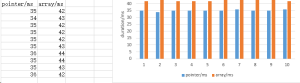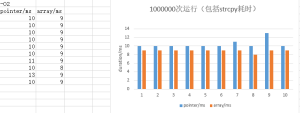# 指针和数组，到底哪个快，顺便讨论写实验报告1个月又10天以前，MYL同学给我看了2段代码，是C语言的字符串接接函数strcat的实现。一段代码用的方法是数组，另一段用的是指针。问题是，一般使用指针实现，而不用数组，是因为指针更快呢，还是仅仅出于习惯/习俗/惯用法。

: void str_cat_pointer(char* from, char* to)
: {
:     while(*to != 0)
:         to++;
:     while(*from != 0)
:         *to++ = *from++;
:     *to=0;
:
: }

void str_cat_array(char from[], char to[])
{
int i,j;
for(i=0; to[i]!= 0; i++)
;
for(j=0; from[j]!=0; i++,j++)
to[i] = from[j];
to[i]=0;
}

1. 准备材料，两个数组/指针字符串。

:     char f=”0123456789″;
:     char t=”abcd”;

2. 操作

:         for(int i=0; i<1000000L; i++)
:         {
:             strcpy(f, “0123456789”);
:             strcpy(t, “abcd”);
:             str_cat_pointer(f, t);
:             //printf(“\n%s\n”, t);
:         }

3. 测量

:     clock_t begin, duration;
:         begin = clock();

:         duration = clock() – begin;
:         printf( “\n%d ms in str_cat_pointer.”, duration*1000 / CLOCKS_PER_SEC );

4. 多测几次

:     for(int k=0; k<10; k++)

5. 对比6. 反转

： “c:\Program Files (x86)\CodeBlocks\MinGW\bin\mingw32-gcc.exe” point.c

： “c:\Program Files (x86)\CodeBlocks\MinGW\bin\mingw32-gcc.exe” -O2 point.c7. 未尽事项

/*
>”c:\Program Files (x86)\CodeBlocks\MinGW\bin\mingw32-gcc.exe”  point.c

>”c:\Program Files (x86)\CodeBlocks\MinGW\bin\mingw32-gcc.exe”  -O2 point.c
*/

#include <stdlib.h>
#include <stdio.h>
#include <time.h>
#include <string.h>

void str_cat_pointer(char* from, char* to)
{
while(*to != 0)
to++;
while(*from != 0)
*to++ = *from++;
*to=0;

}

void str_cat_array(char from[], char to[])
{
int i,j;
for(i=0; to[i]!= 0; i++)
;
for(j=0; from[j]!=0; i++,j++)
to[i] = from[j];
to[i]=0;
}

int main()
{
clock_t begin, duration;

char f=”0123456789″;
char t=”abcd”;

for(int k=0; k<10; k++)
{
//—————————
begin = clock();
for(int i=0; i<1000000L; i++)
{
strcpy(f, “0123456789”);
strcpy(t, “abcd”);
str_cat_pointer(f, t);
//printf(“\n%s\n”, t);
}
duration = clock() – begin;
printf( “\n%d ms in str_cat_pointer.”, duration*1000 / CLOCKS_PER_SEC );
}

for(int k=0; k<10; k++)
{
//—————————
begin = clock();
for(int i=0; i<1000000L; i++)
{
strcpy(f, “0123456789”);
strcpy(t, “abcd”);
str_cat_array(f, t);
//printf(“%s\n”, t);
}
duration = clock() – begin;
printf( “\n%d ms in str_cat_array.”, duration*1000 / CLOCKS_PER_SEC );
}

return 0;
}